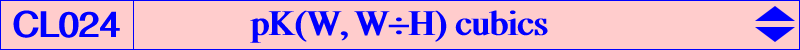Let P and Q be two points in the triangle plane. Pa, Pb, Pc are the projections of P on the lines QA, QB, QC and Qa, Qb, Qc those of Q on the lines PA, PB, PC. The lines PaQa, PbQb, PcQc concur (i.e. the triangles PaPbPc and QaQbQc are perspective) if and only if P lies on a pK invariant under the isoconjugation f which swaps P and Q and whose pivot U is the isoconjugate of the orthocenter H (this solves and generalizes a question raised by Antreas Hatzipolakis). Jean-Pierre Ehrmann observes that this condition is realized if and only if A, B, C, P, Q lie on a rectangular hyperbola - in this case, the lines concur at the pole of the line PQ with respect to the hyperbola. Thus, the lines PaQa, PbQb, PcQc concur if and only if the line PQ goes through f(H). H is obviously a point on the cubic and the tangents at A, B, C are the altitudes of ABC. Naturally, the polar conic of H in the cubic is the rectangular circum-hyperbola passing through U. When U = X(3), we obtain the McCay cubic K003 and when U = X(69), we obtain the Lucas cubic K007. The only circular cubic of this type is Kn = K060. With U = (u : v : w), the barycentric equation is : ∑ SA v w x^2 (SB y – SC z) = 0. *** Another description of this type of pK is the following. Let (C) be the circum-conic with perspector W and denote by A', B', C' the "second" common points of the lines AP, BP, CP and (C). A'B'C' and the pedal triangle of P are perspective if and only if P lies on pK(W, W÷H), together with the circumcircle and the line at infinity. When W = K, (C) is the circumcircle and A'B'C' the circumcevian triangle of P. This is property 6 in K003, the McCay cubic. When W = G, (C) is the Steiner ellipse and we find property 9 in K007, the Lucas cubic. (C) is a rectangular hyperbola if and only if W lies on the orthic axis and then the pivot U lies at infinity. In this case, the cubic has always three real asymptotes : one is the line UH, the two other are parallel to the asymptotes of (C) at the antipode X of H on (C). X is the fourth common point of (C) and the circumcircle i.e. the trilinear pole of the line WK. See for example K245, K246, K247. The cubic pK(W, W÷H) passes through a given point P = p : q : r if and only if W lies on the circum-conic whose perspector is Q = f(P) = p^2(SB q - SC r) : : . In this case, the cubic also passes through the H-crossconjugate of P. Note that Q lies at infinity if and only if P lies on the Lucas cubic K007. The following table gives a selection of such pKs with centers denoted i for X(i).pivot U pole W cubic X(i) on the cubic for i = / remarks 1 19 1, 4, 1148 2 4 K663 2, 4, 253, 1249 3 6 K003 McCay 1, 3, 4, 1075, 1745, 3362 / the only equilateral of the class 4 393 cubic decomposed into the altitudes 5 53 6 25 4, 6, 1988, 3168 7 278 8 281 9 33 10 1826 19 1096 20 1249 21 1172 25 2207 31 1973 32 1974 40 2331 41 2212 42 2333 48 31 63 1 4, 7, 9, 40, 63, 189, 366 / isogonal transform of K145 68 2165 K690 4, 68, 485, 486, 637, 638 69 2 K007 Lucas 2, 4, 7, 8, 20, 69, 189, 253, 329, 1032, 1034, 5932 / (C) is the Steiner ellipse 75 92 76 264 77 57 1, 4, 7, 77, 174, 189, 223 78 9 1, 4, 8, 78, 188, 1034, 1490 95 275 2, 3, 4, 95 184 32 4, 6, 184, 1988 / isogonal transform of K146 264 2052 K647 2, 4, 92, 253, 264, 273, 318, 342, 2052, 7020 265 1989 K060 Kn 4, 5, 13, 14, 30, 79, 80, 265, 621, 622, 1117, 1141, 5627, 6761 / the only circular cubic of the class 286 4 x 286 4, 7, 27, 29, 92, 189, 273, 286, 1896 287 98 K777 2, 4, 6, 98, 253, 287, 290, 325, 401, 1503, 1975, 1988, 9476 296 1945 1, 4, 65, 296, 1935, 1936, 1937, 2655 305 76 2, 4, 75, 76, 253, 305, 341, 1088, 1370 328 94 2, 4, 94, 253, 311, 328, 1141, 3153 394 3 4, 6, 69, 394, 1032, 1498, 1988 520 647 K246 4, 520, 2574, 2575 / (C) is the Jerabek hyperbola 521 650 K247 4, 521, 3307, 3308 / (C) is the Feuerbach hyperbola 525 523 K245 4, 525, 3413, 3414 / (C) is the Kiepert hyperbola 577 184 3, 4, 6, 577 3519 2963 4, 17, 18, 633, 634 / isogonal transform of K349 14841 14840 K194 4, 1656, 1657 14843 14842 K195 4, 3090, 3529Note 1 :The cubics with pivots highlighted in orange are members of the pencil generated by K003 and K007. This pencil also contains the union of the altitudes and, apart this decomposed cubic, all the cubics are tangent at H to the line passing through X(51). The pole lies on the circum-conic passing through X(2), X(6) and the pivot lies on the Jerabek hyperbola. Note 2 :The cubics with poles highlighted in green pass through X(2) and X(253). Their pivots lie on the circum-conic with perspector X(525), passing through X2, X69, X95, X253, X264, X287, X305, X306, X307, X328, X1441, X1494, X1799, X1972, X2373, X2419, etc.